# Module 9: The basic psychrometric processes

The CPD articles in the April and August editions of the CIBSE Journal have considered the main properties of moist air that may be determined from a pair of coincident properties using the Psychrometric Chart and how values may be calculated in the absence of a chart.
This article moves on to develop the core processes that are plotted on the chart as means of illustrating and investigating the changes that take place to the properties of humid air as it is ‘conditioned’. Symbols are defined in the box at the end of the article – you may also find it useful to be able to refer to those earlier articles.

The version of this module in the October edition of CIBSE Journal omitted the options for question one. We apologise for this.
Please note that the options for this question are: A. Figure 2 B. Figure 3 C. Figure 4 D. Figure 5 E. Figure 6
If sending in the tear-out page from the magazine, just write in one of these options under question one.

### The start of the process

The outline of the chart is as shown in Figure 1 and it may be used for individual rooms or processes as well as describing the changes to properties that take place in a complete air handling system. When illustrating the air condition in a project or job notes it might be clearer to refer to a printed chart (possibly conveniently plastic coated and reusable) to read off the data and then convert that into a sketch similar to that shown in the figure where the data and processes are not lost in the plethora of printed lines. When considering whole processes on a standard CIBSE Psychrometric chart (particularly when considering ‘summer’ cycles) the individual process lines can be quite tightly bunched and difficult to discriminate. A photo-enlarged chart (or a specific area from the chart produced by computer software) will improve the resolution.

### Plotting the air point

When lines are drawn on the chart they reflect changes in sensible heat or latent heat or a combination of the two. Sensible heat is that added to, (or taken from), moist air without a change in moisture content and latent heat is that added to, (or taken from), moist air without a change of dry bulb temperature. The energy contained in the air (as indicated by Enthalpy, h) relating to both the sensible and latent energy increases towards the right and the top of the chart as each of dry-bulb temperature and moisture content increase. When using the Psychrometric Chart for plotting cycles that include both latent and sensible change the individual processes are simplified to straight lines. The lines that join the start and end points on ‘real world’ processes that include latent heat transfer may curve; however, for HVAC analysis it is normally just the start and end points that are important.

Sensible heating is shown in Figure 2. This may be achieved, for example, with a heater battery made up from rows of hot water or steam coils, or electric resistance heaters or as the result of some heat recovery mechanism (such as a flat plate heat recovery device). The opposite process, sensible cooling, is shown in Figure 3. For a cooling coil to provide sensible cooling only (and no latent cooling) the coil surface temperature must be greater than the dew-point temperature of the air.

In HVAC it is unlikely that a simple ‘latent’ only cooling process will be present. However ‘combined’ latent and sensible cooling will regularly take place in the same piece of equipment – for example in a cooling coil that has a surface temperature below the dew-point temperature of the incoming air. This combined process will produce an off coil temperature somewhat higher than that of the actual temperature of the coil (known as the Apparatus Dew Point, ADP and shown as point X on Figure 5).

How close the dry-bulb temperature of point B approaches the ADP will depend on the effectiveness of the cooling coil. This will relate such parameters as the number and shape of coil rows, the spacing and type of fins on the coil, and the air velocity.

This is commonly combined in the term known as ‘contact factor’, β – where the contact factor is a measure of how close the air will get to the coil ADP and can be seen as the fraction of the distance between points A and X of the distance A to B ie: contact factor = AB/AX.

By virtue of the way that ‘similar triangles’ work AB/AX = (gA – gB)/ (gA – gX) = (θA – θB)/ (θA – θX). The term ‘bypass factor’ is sometimes used and is simply (1 – contact factor).

The process that would typically result from a steam humidifier (almost pure latent heating) is indicated in Figure 4 – it can reasonably be considered as a vertical line following a constant dry-bulb temperature. A steam humidifier will produce a small amount of sensible heating since the higher temperature of the device (the tube) carrying the steam into the air will increase the temperature of the air and there will be a very slight sensible heating effect from the steam itself.

So-called Adiabatic (constant energy) Humidification as shown in Figure 6 practically characterises the processes of atomising spray and ultrasonic humidifiers (as well as the rarely used recirculating spray and wetted pad humidifiers). By convention the process is shown as being along the wet bulb temperature line (for discussions on the validity of this see Chapter 3 of Jones’ Air Conditioning Engineering book). The concept of a ‘contact factor’, in this case known as saturation efficiency is still apparent as being the fraction of the approach to the saturation curve.

Where two air streams combine adiabatically (as they normally would) the resulting air will have a psychrometric state that is related to the proportions of each of the combining air mass flowrates. This is indicated in Figure 7 as air stream A mixing with air stream B to produce mixed air at condition M. The ratio of the lengths AM to BM is simply the same ratio as the quantities of air mass flowrates (and in this particular case would indicate that the greater mass flowrate is from branch B, ṁB).

The resulting psychrometric states may therefore be determined as, for example, ṁM θM = ṁA θA + ṁB θB and ṁM gM = ṁA gA + ṁB gB

### Process Calculations

To explore the calculations associated with the processes discussed so far consider the cooling coil shown in Figure 8, where all the data has been read off a CIBSE Psychrometric chart. The psychrometric values were based on the on-coil and off-coil dry-bulb and wet-bulb temperatures as indicated in the diagram. For any type of psychrometric process the rate of energy transfer to or from the air may be simply determined from the chart using the relationship Power (kW) = mass flow rate (kg/s) × enthalpy change (kJ/kg) = ṁΔh

So P = 0.75 × (66.0 – 40.5) = 19.1kW

This may be split into the power used to provide the sensible and latent cooling and this is given by ṁ(hY – hB) and ṁ(hA – hY) respectively.

For the same system the Contact Factor, β may be established from β = (θA – θB) / (θA – θX) = (30 – 16.5) / (30 – 10) = 0.67 (or 67 per cent)

and the Bypass Factor will be (1 – 0.67) = 0.33.

The rate of water vapour removed from the air may be obtained from

ṁ × Δg = 0.75 × (0.0140 – 0.0098) = 0.0032 kg/s (or 3.2 g/s)

These calculations may be reorganised to determine unknown variables for any of the processes illustrated in this article. A future article will combine these processes to examine system psychrometry.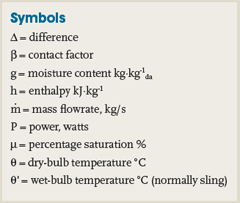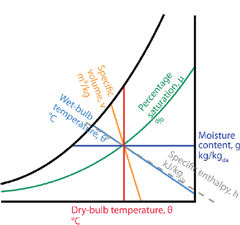Figure 1 – Outline Psychrometric ChartFigure 2 – Psychrometry of Sensible Heating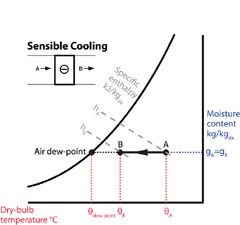Figure 3 – Psychrometry of Sensible Cooling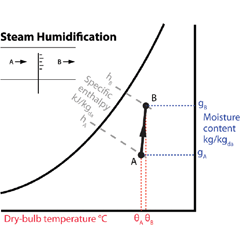Figure 4 – Psychrometry of Steam Humidification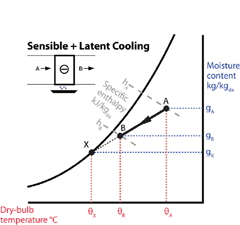Figure 5 – Psychrometry of Sensible and Latent Cooling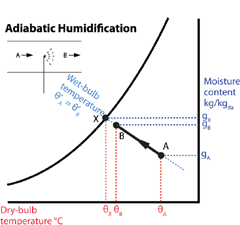Figure 6 – Psychrometry of Adiabatic Humidification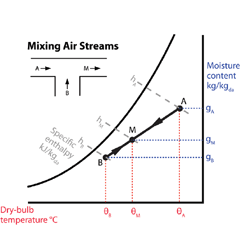Figure 7 – Psychrometry of Mixing Air Streams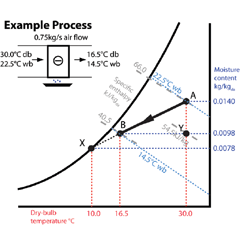Figure 8 – Example Psychrometric Process